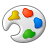# Setting attribute values through CGA rules

1102
1
06-13-2017 04:43 PMNew Contributor

Hi,

There is probably a very simple answer to this question but I'm having a difficult time figuring out how to set it up. I would like to be able to set attribute values for multiple buildings at once using a CGA rule file, rather than manually selecting every relevant model and changing values in the Inspector window.

As two very basic starter examples I was trying, I am looking at three attributes: height, area, and score/volume. I already have the height and area values associated with each building, and I would like to create a rule file/rule files that (1) assign certain values (score) to certain buildings, and (2) assign mathetmatically calculated values (volume) to buildings. The score and volume fields were created but are currently empty (i.e. value zero).

Starting with Score, I tried to very simply set up a rule file that would assign a value of 1 to buildings with a greater height than area, a score of 2 to buildings with equal height and area, and a value of 3 to buildings with a greater area than height. I tried using the script below but the score values remained at zero.

attr height = 0
attr area = 0
attr score = 0

Lot --> extrude(height) Mass

Mass -->
case height > area :
set(score, 1)
case height == area :
set(score, 2)
else :
set(score, 3)

I also tried the variation below, which also did not work.

attr height = 0
attr area = 0
attr score = 0

Lot --> extrude(height) Mass

Mass -->
case height > area :
score = 1
case height == area :
score = 2
else :
score = 3

I'm sure it is a simple fix but I'm not sure which grammar to use. I also wanted to do one that sets the attribute value for volume by calculating (height x area), but I don't think I would be able to get that one to work if I can't get this one to work. Any help would be much appreciated!

Thank you,

kmbyEsri Regular Contributor

In the first piece of code, the score is actually being set in the rules, but it's only being set in the Mass rule.  Attributes are initialized before any rules are executed, and these are the values that you see in the Inspector.  So, score is set to 0 in the Inspector.  Then, as we progress through the rules, we first come across Lot which generates a Mass shape in the shape tree.  So, for the shape Lot and the shape Mass in the shape tree (to see the shape tree:  Window -> Show Model Hierarchy), the value of score is 0.  In the Mass rule, the attribute score is set to 1, 2, or 3, and so, for the shapes that come after Mass (in this case the implicit terminal shape which is also called Mass) the attribute score has a non-zero value, but this is something you don't see in the Inspector because it is a value that is set for only part of the shape tree.

In the second piece of code, the syntax score = 1 is not allowed.  This won't compile.

I assume that the attributes height and area are getting values from linked object attributes, and that's why they are not zero.

You can calculate score and volume when you define these attributes like this:

``````attr score =
case height>area:  1
case height==area: 2
else:              3

attr volume = height*area‍‍‍‍‍‍``````

The first approach is not wrong though.  You could also set attributes values inside the rules.  Just know that you won't see these values in the Inspector.  This approach is helpful when you want to set different values on different parts of your model.